﻿ An Order Level Inventory Model for Deteriorating Items with Time – Quadratic Demand and Partial Backlogging

### An Order Level Inventory Model for Deteriorating Items with Time – Quadratic Demand and Partial Back...OPEN ACCESSPEER-REVIEWED

## An Order Level Inventory Model for Deteriorating Items with Time – Quadratic Demand and Partial Backlogging

J. Jagadeeswari1,, P. K. Chenniappan2

1Department of Mathematics Sri Ramakrishna Engineering College Coimbatore, Tamilnadu, India

2Department of Mathematics Government Arts College (Autonomous) Coimbatore, Tamilnadu, India

### Abstract

In this paper, a deterministic inventory model is investigated for deteriorating items in which the demand is time quadratic and shortages are allowed and partially backlogged. The backlogging rate is assumed to be dependent on the length of the waiting time for the next replenishment. The longer the waiting time is, the smaller the backlogging rate would be. The deterioration rate is assumed to be constant.

• Jagadeeswari, J., and P. K. Chenniappan. "An Order Level Inventory Model for Deteriorating Items with Time – Quadratic Demand and Partial Backlogging." Journal of Business and Management Sciences 2.3 (2014): 79-82.
• Jagadeeswari, J. , & Chenniappan, P. K. (2014). An Order Level Inventory Model for Deteriorating Items with Time – Quadratic Demand and Partial Backlogging. Journal of Business and Management Sciences, 2(3), 79-82.
• Jagadeeswari, J., and P. K. Chenniappan. "An Order Level Inventory Model for Deteriorating Items with Time – Quadratic Demand and Partial Backlogging." Journal of Business and Management Sciences 2, no. 3 (2014): 79-82.

 Import into BibTeX Import into EndNote Import into RefMan Import into RefWorks

### 1. Introduction

In the last three decades, the models for inventory replenishment policies involving time-varying demand patterns have received the attention of several researchers. Researchers considered only two types of time – dependent demands, linear and exponential. Linear time – dependence of demand implies a uniform change in the demand rate of the product per unit time. This is rarely seen to occur in the real market. On the other hand, an exponentially time – varying demand also seems to be unrealistic because an exponential rate of change is very high and the market demand of any product may not undergo such a high rate of change as exponential. An alternative approach is to consider quadratic time – dependence of demand which may represent all types of time – dependence depending on the signs of the parameters of the time – quadratic demand function.

The fundamental result in the development of economic order quantity model with time-varying demand patterns is that of Donaldson (11) who established the classical no-shortage inventory model with a linear trend in demand over a known and finite horizon. However, his procedure was too complex and tedious in computing. The complexity of Donaldson’s approach has led to the development of heuristic methods. Dave and Patel (12) first considered the inventory model for deteriorating items with time-varying demand. They considered a linear increasing demand over a finite horizon and a constant deterioration rate. Sachan (13)extended Dave and Patel’s model to allow shortages.

All researchers have assumed that during shortage period all demand is either backlogged or lost. In practice, some customers would like to wait for backlogging during the shortage period, but the others would not. Consequently, the opportunity cost due to lost sales should be considered.

In this paper, backlogging rate is assumed to be a fixed fraction of demand rate during the shortage period. However, in some inventory system, for many stock such as fashionable commodities, the length of the waiting time for the next replenishment becomes a major factor for determining whether the backlogging will be accepted or not. The longer the waiting time is, the smaller the backlogging rate would be. Therefore, the backlogging rate is variable and is dependent on the waiting time for the next replenishment. Here an attempt has been made to model the situation where the demand rate is a time-quadratic function and the items deteriorate at a constant rate with partial backlogging.

In section 2 the assumptions and notations, which we have followed to derive this model, are clearly given. In section 3 we develop a mathematical model for the situation. Also, an analytical solution is given for the same. In section 4 we have done sensitivity analysis of our model. In section 5 the concluding remarks are given.

### 2. Assumptions and Notations

The mathematical model in this paper is developed on the basis of the following notations and assumptions.

2.1. Notations

A – Ordering cost per order

h – Inventory carrying cost per unit per unit time

s – Shortage cost per unit per unit time

l – Opportunity cost due to lost sales per unit

r – Purchase cost per unit

t1 – the time at which the inventory level reaches zero, t1 ≥ 0

t2 – the length of period during which shortages are allowed, t2 ≥ 0

T – ( =t1 + t2) the length of cycle time

I1(t) – the level of positive inventory at time t, 0 ≤ t ≤ t1

I2(t)-the level of negative inventory at time t, t1tt1 + t2

Θ-Deterioration rate, a fraction of the on-hand inventory

IM – Maximum inventory level during [0, T]

IB – Maximum inventory level during shortage period

R(t) – Demand rate at any time t ≥ 0

2.2. Assumptions

•  A single item is considered with a constant rate of deterioration.

•  The replenishment occurs instantaneously at an infinite rate.

•  A deteriorated unit is neither repaired nor replaced during a given cycle

•  The deterministic demand rate R(t) varies quadratically with time, i.e., R(t) = a + bt + ct2, where a, b and c are constants such that a, b, c> 0.

•  Shortages are allowed and partially backlogged.

•  During stock out period, the backlogging rate is variable and is dependent on the length of the waiting time for the next replenishment. The backlogging rate is assumed to be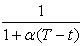where the backlogging parameter α is a positive constant and (Tt) is waiting time (t1t T).

### 3. Mathematical Model

The rate of change of inventory during positive stock period [0, t1] and shortage period [t1, T] is governed by the differential equations(1)(2)

With boundary conditions,

I1(t) = I2(t) = 0 at t = t1 and I1(t) = IM at t = 0.

3.1. Analytical Solution

During the period [0, t1], the inventory depletes due to the deterioration and demand. Hence the inventory level at any time during [0, t1] is described by equation (1) which is a linear ordinary differential equation of first order and its integrating factor is eθt. Multiplying both sides of (1) by eθt and then integrating over [0, t] and applying the boundary condition I1(t1) = 0 we have(3)

During the interval [t1, T] the inventory level depends on demand and a fraction of demand is backlogged. The state of inventory during [t1, T] is represented by the equation (2) and it’s solution is given by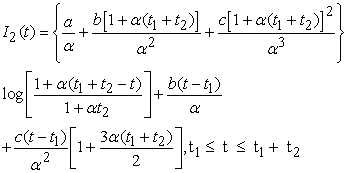(4)

The total cost per replenishment cycle consists of the following components: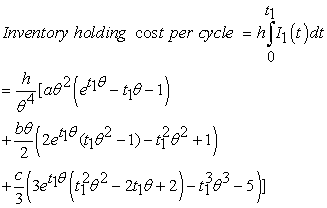(5)(6)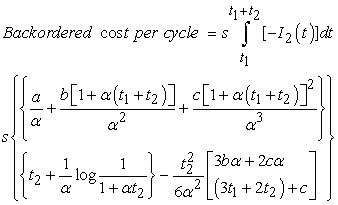(7)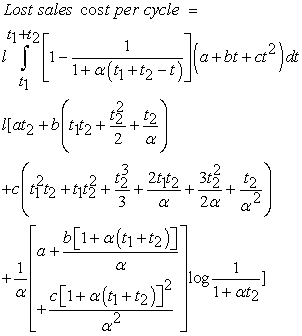(8)

When t = 0 the level of inventory is maximum and it is denoted by IM. Then from equation (3)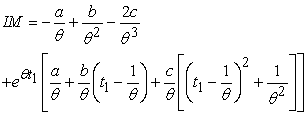(9)

The maximum backordered inventory is obtained at t = t1 + t2 and is denoted by IB. Thus from equation (4)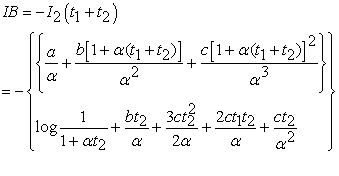(10)

Thus the order size during total time interval (0, T) is given by(11)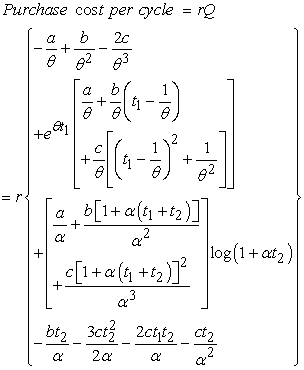(12)

Therefore the Total cost (TC) per time unit is given by,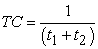[Ordering cost + Inventory holding cost + Cost of deterioration + Backordered cost + Lost sales cost + Purchase cost](13)

The necessary condition for the total cost per time unit to be a minimum is### 4. Sensitivity Analysis

Consider an inventory system with the following parameter in proper units:

A = 250, h = 0.05, s = 1.2, l = 1.5, α = 8, θ = 0.005, a = 25, b = 20, c = 15. The computed optimal value of TC is 92.53. The variation in the parameters is as follows:

#### Table 4.5. Variation in parameter a

From tables 4.1, 4.2, 4.3, 4.4 and 4.5 it is observed that the total cost increases if the parameters α, θ, a, b and c are increased. Also the parameters a, b and c are more sensitive than α and θ.

### 5. Conclusion

In this paper, we have developed an order quantity model for deteriorating items with time – quadratic demand and partial backlogging. In particular, the backlogging rate is considered to be a decreasing function of the waiting time for the next replenishment. This assumption is more realistic in the market because customers generally do not wait for longer period for service.

### References

  Silver, E.A., Pyke, D.F., and Peterson, R., 1998, Inventory Management and Production Planning and Scheduling, John Wiley and Sons, New York.In article  Raafat, E., 1991, Survey of Literature on continuously deteriorating inventory model, Journal of Operational Research Society, 42, 27-37.In article CrossRef  Chang H.J. and Dye C.Y., 1999, An EOQ model for deteriorating items with time varying demand and partial backlogging, Journal of Operational Research Society, 50, 1176-1182.In article CrossRef  Goyal, S.K., and Giri, B.C., 2001, Recent trends in modeling of deteriorating inventory, European Journal of Operational Research, 134, 1-16.In article CrossRef  Teng, J. T. and Chang, C. T., 2005, Economic production quantity models for deteriorating items with price and stock dependent demand, Computers and Operational Research, 32, 297-308In article CrossRef  S.R. Singh and T.J. Singh. 2007, An EOQ Inventory model with Weibull Distribution deterioration, Ramp type demand and Partial Backlogging, Indian Journal of Mathematics and Mathematical Sciences, 3 (2), 127-137.In article  T.J. Singh, S.R. Singh and R. Dutt, 2009, An EOQ model for perishable items with power demand and partial backlogging, International Journal of Production Economics, 15 (1), 65-72.In article  You S.P., 2005, Inventory policy for products with price and time dependent demands, Journal of Operational Research Society, 56, 870-873.In article CrossRef  Pareek S., Mishra V. K and Rani S., 2009, An Inventory model for time dependent deteriorating item with salvage value and shortages, Mathematics today, 25, 31-39.In article  Hamid Bahari, 1989, Replenishment schedule for deteriorating items with time proportional demand, Journal of Operations Research Society, 40, 75-81.In article CrossRef  Donaldson, W. A, 1979, Inventory replenishment policy for a linear trend in demand-an analytical solution, Operational Research Quarterly, 28, 663-670.In article  Dave, U. and Patel, L. K, 1981, (T, Si) policy inventory model for deteriorating items with time proportional demand, Journal of Operational Research Society, 32, 137-142.In article  Sachan, R. S, 1984, On (T, Si) inventory policy model for deteriorating items with time proportional demand, Journal of Operational Research Society, 35, 1013-1019.In article  Lin C., Tan B. and Lee W.C., 2000, An EOQ model for deteriorating items with time-varying demand and shortages, International Journal of System Science, 31 (3), 390-400.In article CrossRef  Hariga M., 1996, Optimal EOQ models for deteriorating items with time-varying demand, Journal of Operational Research Society, 47, 1228-1246.In article CrossRef